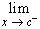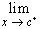#Interactive Real Analysis

Next | Previous | Glossary | Map

## 6.1. Limits

### Definition 6.1.8: One-Sided Limits of a Function

If f is a function with domain D and cclosure(D). Then:
• f has a left-hand limit L at c if for every> 0 there exists> 0 such that if xD and c -< x < c then | f(x) - L | <. We writef(x) = L
• f has a right-hand limit L at c if for every> 0 there exists> 0 such that if xD and c < x < c +then | f(x) - L | <. We writef(x) = L
Next | Previous | Glossary | Map